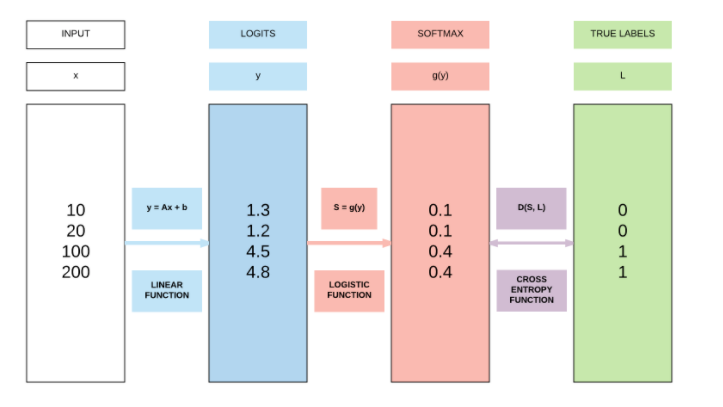# Activation functions — Why the need?

Original article was published by Dipanwita Mallick on Deep Learning on Medium# Step function

Essentially, it means if the input is greater or equal to a threshold, then the output will be 1, meaning the neuron will be activated else not. NOT used often.

# Sigmoid function

What this function does is — takes the input and outputs a probability value that obviously lies between 0 and 1. And for that reason it is mostly used in the last layer of binary classification.

# TanH function

The hyperbolic TanH function is nothing but the scaled sigmoid function and little shifted so it outputs a value that lies between +1 and -1. Mostly used in the hidden layers.

# ReLU function

The ReLU activation function is widely used in the hidden layers and is one of the most popular choices. What it does is — it outputs 0 for negative input values and simply outputs the input for positive values. Now, it might seem confusing, but it is a non-linear transformation.

# Leaky ReLU function

Leaky ReLU is an improvement over ReLU and tries to solve a problem called- vanishing gradient. How is it similar to ReLU ? It is similar in a way that for inputs greater that 0 the output will be the input (like ReLU) but for inputs less than 0, the output will not be 0 but rather the input multiplied with a very small number say 0.0001. How does it help? For ReLU, since the values are zero for inputs less than 0, the gradients will also be zero in back propagation, meaning there will be no weight updation and the neurons will stop learning. So, when the weights are seen not updating then it’s a good idea to use Leaky ReLU in the hidden layers.

# Softmax function

Softmax function is a popular choice for the last layer of a multi-class classification problem. It takes the inputs and outputs them as probability values, meaning the outputs will lie between 0 and 1.

Conclusion: With this I will end my introduction on activation functions. This write up can be used as a quick reference to activation functions during any interview prep or in general while working on deep learning 🙂